# Upsilon background over events update

## Normalisation

The other week when I showed this analysis I was uisng a simple method of normalisation, i.e intergrate in a region that avoided the upsilon peak (the N+- plots) and normalise the background over events to this Previous analysis.

Now I am using a method as follows the NA50 groups Dilepton analysis, normalise the background over event spectra to the same event paired background as the right hand side of:

ratio = ( Bg mixed )/(Sig) = (Bg mixed)/(2 sqrt(N++*N--) )

Because if we assume a perfect combinotorial background sig=(2 sqrt(N++*N--) ) (The real event background)

This method allows us to normalise without referring to real event Signal (N+-) spectra, but to the real event Background (N++ and N--). We normalise over the whole range of the N++ and N-- spectra, as there is no signal. However, note that this is not exact for the "background over events". We are infact shifting this spectra up and down, keeping the shape, to match the exact normalisation from N++/N--

N++ vs. normalised "Background over event"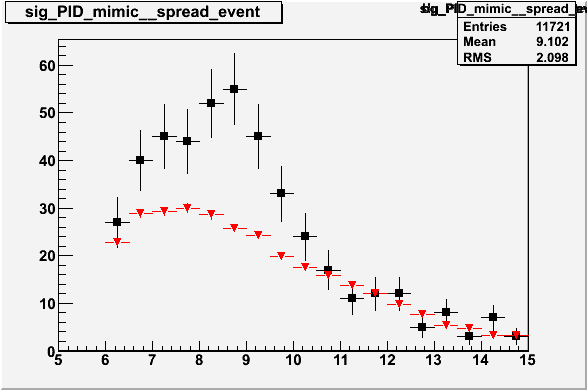## Subtracted spectra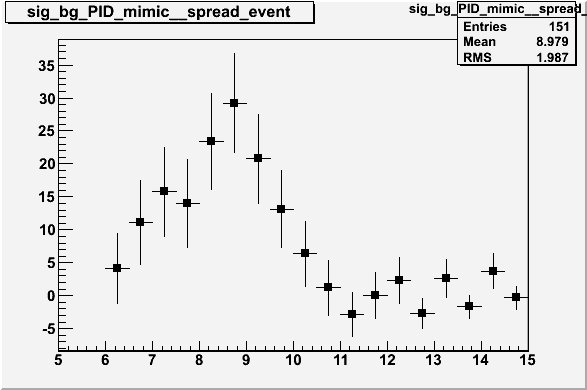# QA

The normalisation seems to be too low, it was pointed out by Saskia that as we are mixing on data that has passed the mimic trigger and that we may be putting a bias into the "Events over Background" (EOB) distribution. This happens because we find more L0-L0 combinations when we pair tracks from more than one event, which each have an L0 trigger, than when we pair within the same event.

## Here is the ratio of the EOB to N++/N-- (real background) mass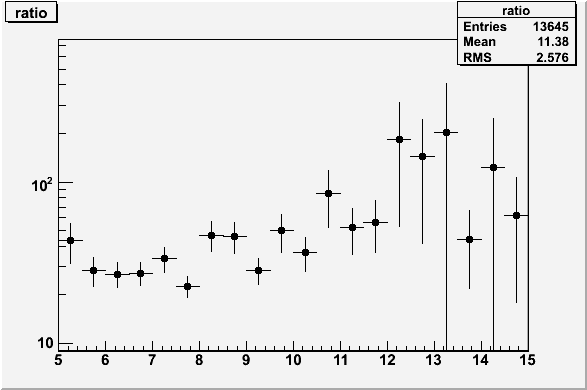It can be seen that the distribution is not flat

If the Bias was coming from the trigger we would expect the energy distributions for the paired tracks to differ between the N++/N-- and the EOB case. To see this I took the average energy for a pair and plotted the ratio EOB to N++/N-- (ratio of mixed bg to real background)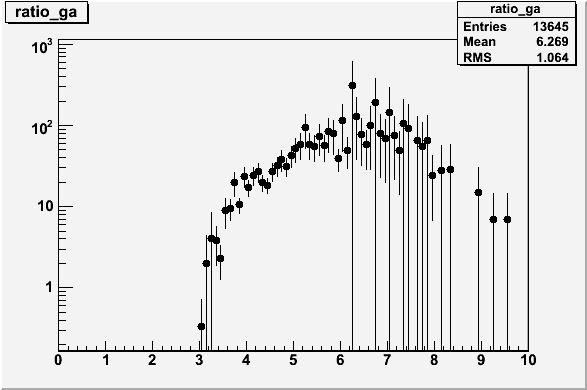Here we see some clear trend where the EOB is not consistant with the N++/N-- real background

# Removing the High track

This method looked at what happend to the EOB distribution if we never mixed the higest track energy in the real-event background with the highest track in each of the EOB (mixed) event-backgrounds. This should stop the excess of L0/L0 triggers being generated in the EOB distribution

## With Hi energy mixing removed, EOB/N++N-- ratio mass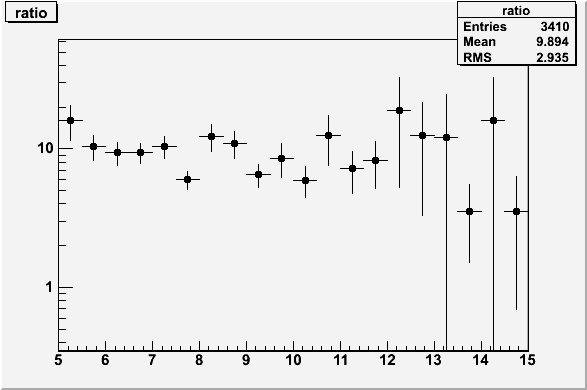## With Hi energy mixing removed, EOB average pair eng./N++N-- average pair eng.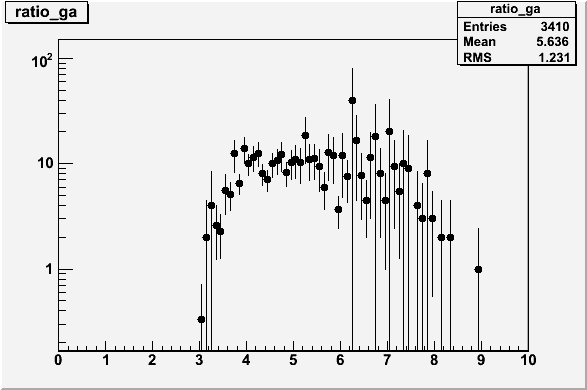# Signal

 No Hi energy combination cut (Sig vs Bg.) With Hi enegy combination cut (Sig vs Bg.)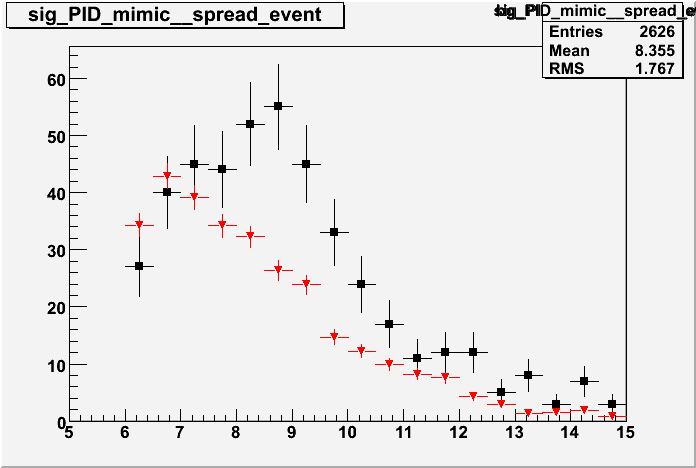# Hi energy mixing mass

### Fit value: Mean=9GeV, Width +/- 1GeV, Sum of BINS within this range = 97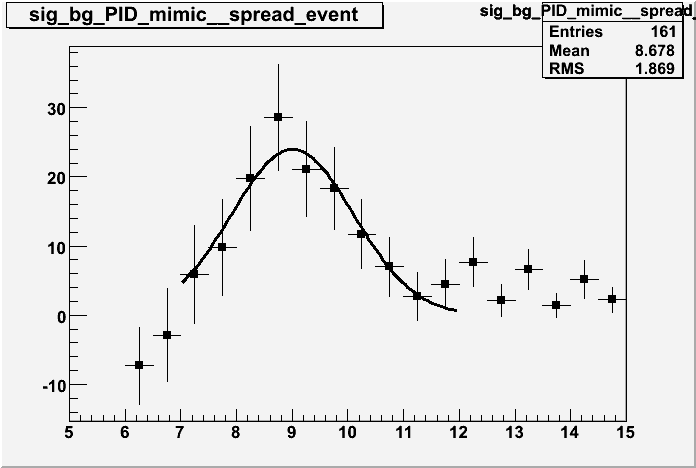### Fit value: Mean=9.7GeV, Width +/- .7GeV, Sum of BINS within this range = 68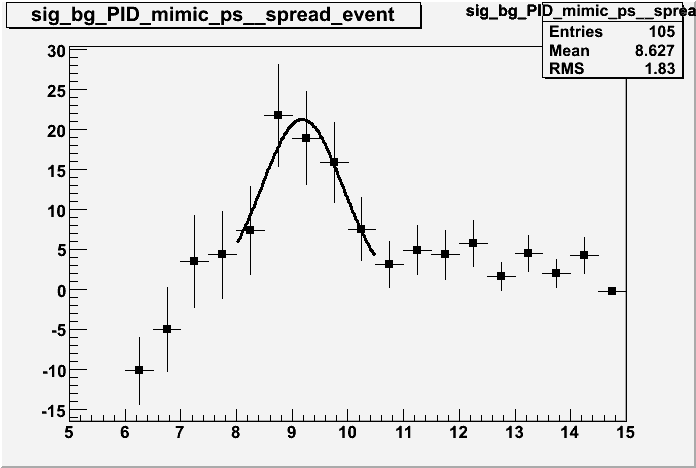# Conclusion

The preshower is about 70% efficient

(See Hi energy ratio plot) At this stage it seems that the EOB is losing events at energies under the L0 4.5 GeV trigger level and distorting the distribution and this is where I will be focusing next